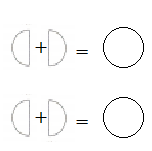Refer to our Texas Go Math Grade 1 Answer Key Pdf to score good marks in the exams. Test yourself by practicing the problems from Texas Go Math Grade 1 Lesson 16.2 Answer Key Halves.

Explore

Draw to solve.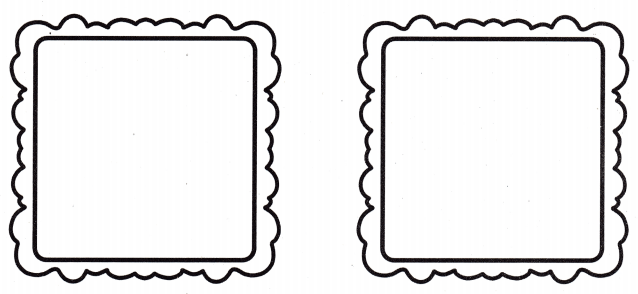FOR THE TEACHER • Have children draw to solve this problem: Two friends share the sandwich on the left. How can they cut the sandwich so each gets an equal share? Then have children solve this problem: Two other friends share the sandwich on the right. How could this sandwich be cut a different way so each friend gets an equal share?Explanation:
Two friends share the sandwich on the left, so the left side sandwich has to be cut into two equal parts
And, Two other friends share the sandwich on the right so the right side sandwich has also to be cut into two equal parts

Math Talk
Mathematical Processes

Will all four friends get the same amount of sandwich?
Answer: Yes all four friends will get the same amount of sandwich as first two friends share the sandwich on the left, so the left side sandwich has to be cut into two equal parts
And, Two other friends share the sandwich on the right so the right side sandwich has also to be cut into two equal parts

Model and Draw

The 2 equal shares make 1 whole.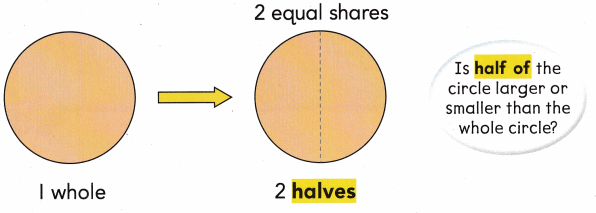Share and Show

Draw a line to show halves.

Question 1.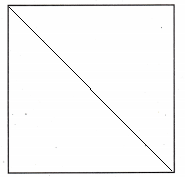Question 2.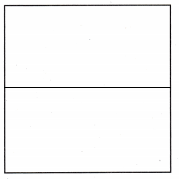Question 3.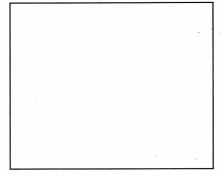Question 4.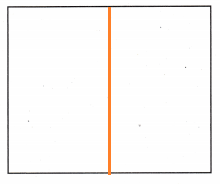Problem Solving

Name the parts. Write halves or not halves.Question 5.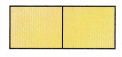Question 6.Question 7.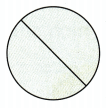Question 8.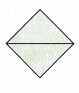Question 9.Question 10.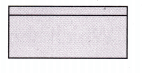Question 11.
H.O.T. Use the picture. Write numbers to solve.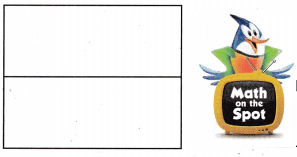The picture shows ______ halves.
The ______ equal shares make whole.The picture shows two halves.
The two equal shares make  a whole.

Question 12.
H.O.T. Multi-Step Draw three different ways to show halves.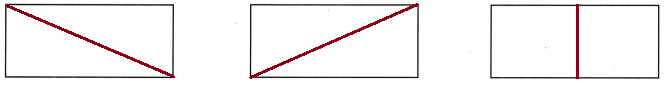Question 13.
Sandy has a whole sandwich. She shares an equal part with her friend. Which shows halves?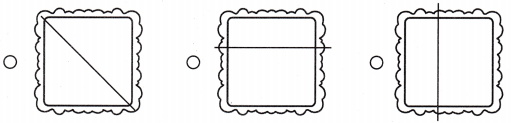Question 14.
Which shape does not show halves?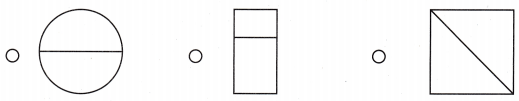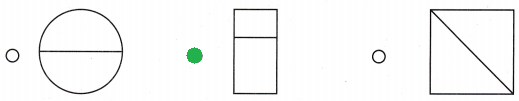Question 15.
Reasoning Useto help you. Kevin has three halves of a circle. How many whole circles does Kevin have?
(A) 1
(B) 3
(C) 2Explanation:
There are three halves of a circle
Two halves makes one whole
so, Kevin will have one whole circle and a half

Question 16.
Texas Test Prep Which shows halves?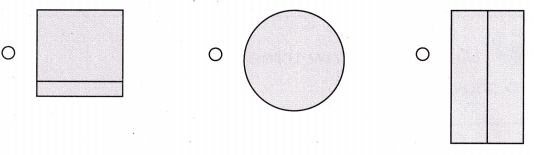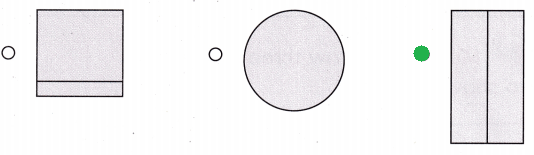TAKE HOME ACTIVITY • Draw a rectangle on a piece of paper. Ask your child to draw o line to show halves.### Texas Go Math Grade 1 Lesson 16.2 Homework and Practice Answer Key

Draw a line to show halves.

Question 1.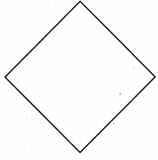Question 2.Question 3.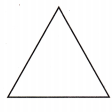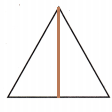Question 4.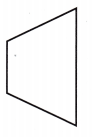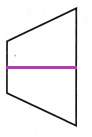Problem Solving

Multi-Step Draw three different ways to show halves.

Question 5.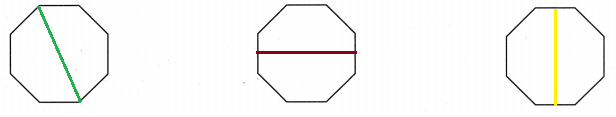Question 6.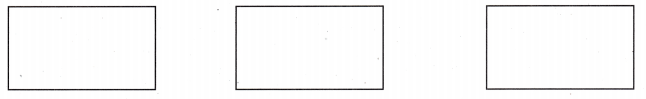Lesson Check

Question 7.
Becca has a dog cookie for her dogs Daisy and Champ. Which shows halves?Question 8.
Jose cuts paper hearts into two equal parts. Which shows halves?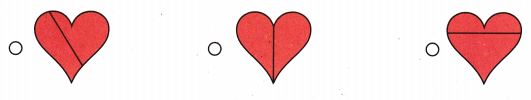Question 9.
Use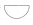to help you. Nia has five halves of a circle. How many whole circles does Nia have?
(A) 2
(B) 1
(C) 3